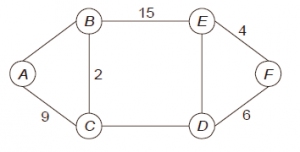# GATE | GATE-CS-2015 (Set 1) | Question 53

• Last Updated : 28 Jun, 2021

The graph shown below 8 edges with distinct integer edge weights. The minimum spanning tree (MST) is of weight 36 and contains the edges: {(A, C), (B, C), (B, E), (E, F), (D, F)}. The edge weights of only those edges which are in the MST are given in the figure shown below. The minimum possible sum of weights of all 8 edges of this graph is ______________.(A) 66
(B) 69
(C) 68
(D) 70

Explanation: In every cycle, the weight of an edge that is not part of MST must by greater than or equal to weights of other edges which are part of MST.

Since all edge weights are distinct, the weight must be greater.

So the minimum possible weight of ED is 7, minimum possible weight of CD is 16 and minimum possible weight of AB is 10.

Therefore minimum possible sum of weights is 69.

Quiz of this Question

My Personal Notes arrow_drop_up